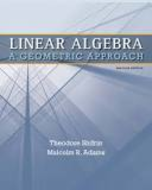Linear Algebra: A Geometric Approach - 2 Edition - Chapter 1.2 - Problem 19
Register Now

Join StudySoup

Get Full Access to Linear Algebra: A Geometric Approach - 2 Edition - Chapter 1.2 - Problem 199781429215213

# a. Give an alternative proof of the Cauchy-Schwarz Inequality by minimizing the

Linear Algebra: A Geometric Approach | 2nd Edition

Problem 19

a. Give an alternative proof of the Cauchy-Schwarz Inequality by minimizing the quadratic function Q(t) = _x ty_2. Note that Q(t) 0 for all t . b. If Q(t0) Q(t) for all t , how is t0y related to x_? What does this say about projyx?

Accepted Solution
Step-by-Step Solution:

Step 1 of 3

To construct an alternative proof for the Cauchy Schwarz inequality.

Let us consider a quadratic function(i).

It is noted thatfor all.

Now,###### Chapter 1.2, Problem 19 is Solved

Step 2 of 3

Step 3 of 3

Unlock Textbook Solution